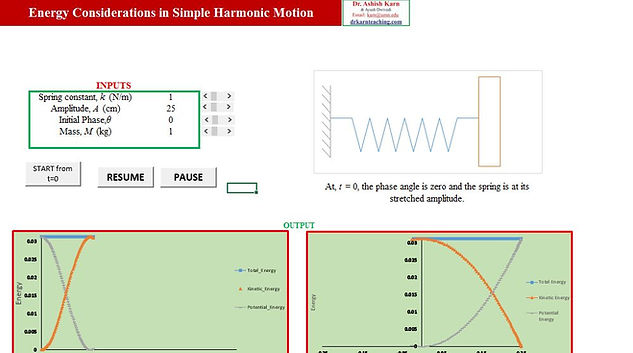top of page
##### K-12 Physics Tools

A running animation of a spring-block system, in sync with plots of kinetic energy, potential energy and total energy both with respect to time-period and displacement of the block are shown. Students can vary mass of the block, initial phase angle, amplitude of oscillation as well as spring constant to study its impact upon the simple harmonic motion. PAUSE and RESUME buttons provide a way to study the phenomenon in greater detail, for self-learning as well as classroom demonstrations.A running animation of a spring-block system with a damper, in sync with plots of total energy and displacement with respect to time are shown. Students can vary mass of the block, initial phase angle, amplitude of oscillation, spring constant as well as damping constant to study its impact upon the simple harmonic motion. STOP and RUN buttons provide a way to study the phenomenon in greater detail, for self-learning as well as classroom demonstrations.This tool helps one to determine the frequency of a string which is fixed at one/both ends by varying the amplitude, length of string, wave speed and the number of harmonic. Upon running the tool, one can study the variation of amplitude and frequency of waves under different conditions.A running animation of a moving SOURCE and a moving OBSERVER is shown along with the frequency and wavelength as recorded by the observer. The students can vary the speeds of the source, observer, speed of sound as well as the source frequency. The source and observer speeds can be set to be negative/positive/zero to cover all cases of stationary/moving source approaching/ receding from a stationary/moving observer. The variation in the observed frequency when the source passes the observer is worth noting.Here is an excellent tool to help students visualize the concepts and calculations of moments of inertia/ radius of gyration about different axes, using parallel and perpendicular axis theorem. Apart from varying the mass and dimensions of the objects, students can choose any axis about which the moment of inertia has to be calculated and a visualization plot aids the student in the correct calculations. Further, these calculations can be done for different shapes such as thin rod, rectangular plate, rings, discs, solid/hollow cylinders and spheres. Click on the title to download.By varying the mass of the body and its location (under the earth's surface/ on the earth's surface/ above the earth's surface), one can get to know the acceleration due to gravity and the weight of the body at the chosen location. It gives an intuitive feeling to the student, as to how much the weight of a body can vary with respect to altitude and depth.